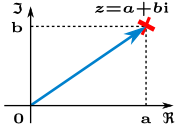×#### Thank you for registering.

One of our academic counsellors will contact you within 1 working day.

Click to Chat

1800-1023-196

+91-120-4616500

CART 0

• 0

MY CART (5)

Use Coupon: CART20 and get 20% off on all online Study Material

ITEM
DETAILS
MRP
DISCOUNT
FINAL PRICE
Total Price: Rs.

There are no items in this cart.
Continue Shopping```Complex Number SystemIndian mathematician Mahavira (850 A.D.) was first to mention in his work 'Ganitasara sangraha'; 'As in nature of things a negative (quantity) is not a square (quantity), it has, therefore, no square root'. Hence, there is no real number x which satisfies the polynomial equation x2+1=0.

A symbol √(-1), denoted by letter i was introduced by Swiss Mathematician, Leonhard Euler to provide solution of equation x2+1=0.'i' was regarded as a fictitious or imaginary number which could be manipulated algebraically like an ordinary real number, except that its square was -1. The letter i was used to denote √(-1), possibly because i is the first letter of the Latin word, 'imaginarius'.

To permit solutions of such polynomial equations, the set of complex numbers is introduced. We can consider a complex number as having the form a + bi where a and b are real numbers. It is denoted by z, i.e., z = a+bi. 'a' is called as real part of j which is denoted by (Re z) and 'b' is called as imaginary part of z which is denoted by (Img). Here, a and b are real numbers.

Complex PlaneThe point ‘z’ represented in red is the complex number z = a+ib which corresponds to an intercept ‘a’ on the x-axis while it corresponds to an intercept ‘b’ on y-axis. Complex number does not actually mean complicated. In fact, it just implies that a complex number is a combination of a real and imaginary number. The line in blue represents the position vector of the point z.

Complex plane is also termed as the Argand plane. A complex number is actually a point or a position vector in a two dimensional Cartesian coordinate system. The real part of the complex number is graphed as the horizontal component while the imaginary part is plotted against the vertical component. When we talk of a position vector of a complex number, it always takes into consideration, the magnitude and the direction with respect to the origin. All this is also emphasized in the polar form.

Some Key Points of Complex Numbers

Complex numbers is an important topic of IIT JEE. Some of the key points of IIT JEE complex numbers are listed below:

As explained, complex numbers are generally expressed as z = a+ib. Hence z is:

Purely real, if b = 0

Imaginary, if b ≠ 0

Purely imaginary, if a = 0

The set R of real numbers is a proper subset of the complex numbers.

Zero is purely real as well as purely imaginary but not imaginary.

i = √(-1), is called the imaginary unit. Also, i2 = -1; i3 = i; i4=1, etc.

√a√b√c  =  √(abc........)

iff at least one of a,b,c ........ is non-negative.

If j = a + ib, then a-ib is called complex conjugate of j.

Real numbers satisfy order relations whereas imaginary order relation, i.e.,

i > 0, 3+i < 2 are meaningless.

Addition and Multiplication of Complex Numbers

Complex numbers are not very different from real numbers except for the fact that they contain imaginary numbers as well. The operations of addition and multiplication are defined in the same way as in the case of real numbers and commutative, distributive and associative properties also hold true. We discuss the addition and multiplication of complex numbers:

Addition of two complex numbers is the same as in real numbers. The real part is added to the real part, while the imaginary parts get added to each other.

(a + ib) + (c + id) = (a + c) + i(b + d)

Multipli9cation of complex numbers is also very simple. Here, every term gets multiplied to every other term and i2 = -1. Using these facts, let us multiply the complex numbers.

(a+ib)(c+id) = a.(c + id) + ib (c + id)

= [ac + aid] + [bic + bi.di]

= ac + adi + bci + bidi

= ac + adi + bci – bd

You may also view the video on complex numbers

askIITians offers comprehensive study material and notes on complex numbers for IIT JEE. All the questions of the JEE have been explained in detail and the material also contains IIT question of complex number with solution. It is important to have a thorough knowledge of the topic in order to remain competitive in the JEE. The Complex numbers study material helps in building a good base.

Related Resources

Look into the Previous Year Papers with Solutions to get a hint of the kinds of questions asked in the exam.

You can get the knowledge of Useful Books of Mathematics here.

To read more, Buy study materials of Complex Numbers comprising study notes, revision notes, video lectures, previous year solved questions etc. Also browse for more study materials on Mathematics here.
```### Course Features

• 731 Video Lectures
• Revision Notes
• Previous Year Papers
• Mind Map
• Study Planner
• NCERT Solutions
• Discussion Forum
• Test paper with Video Solution